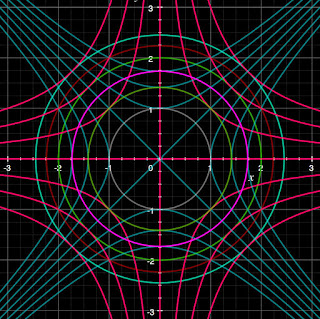### Web of Intriguey^2-x^2 = k
xy = k
k = -3..3
x^2+y^2 = n
n = 1..6

-

Lines, hyperboli, and circles ... oh my!

[Inspired by a figure in Anton's Calculus, 8th Ed.]